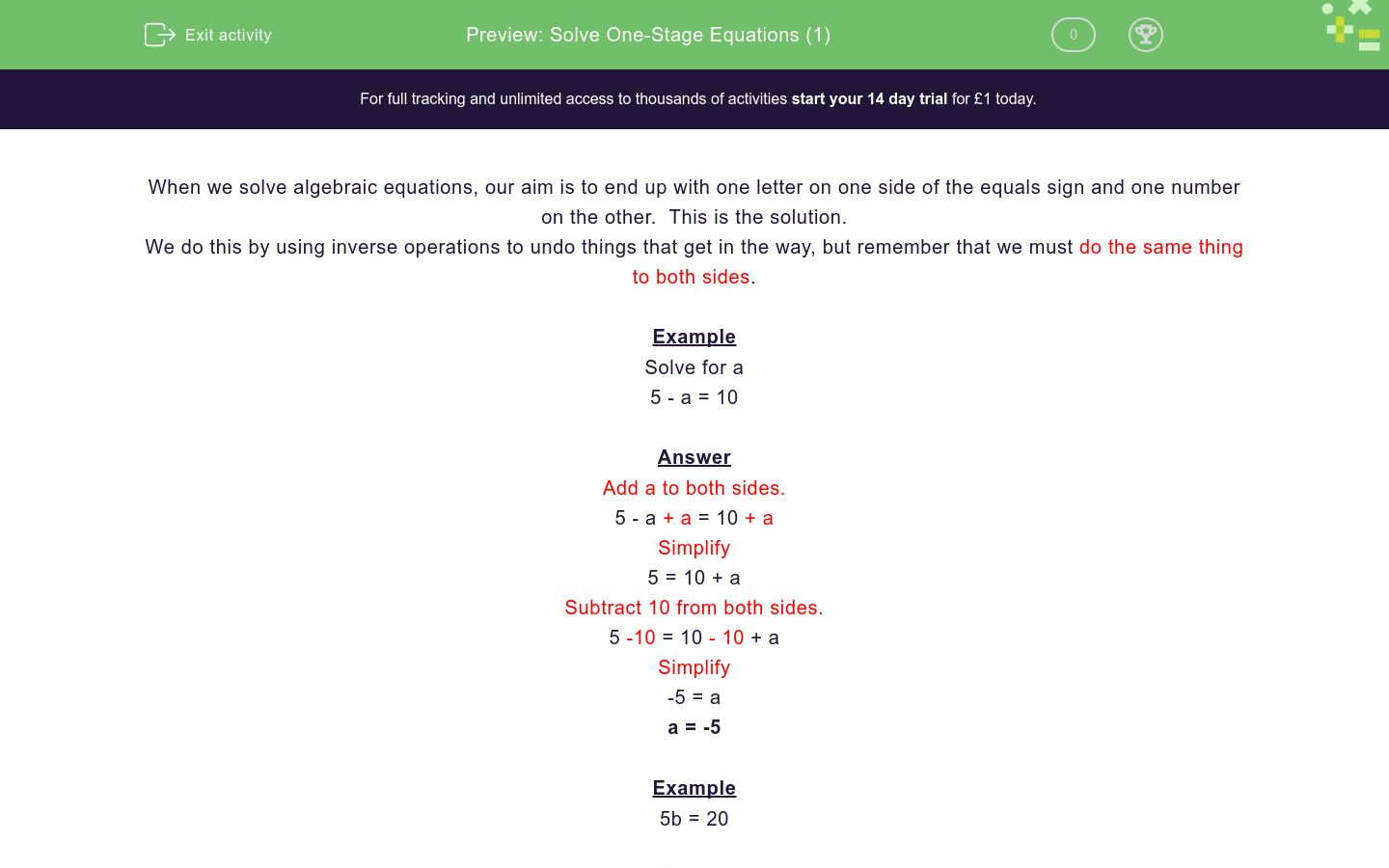# Solve One-Stage Equations (1)

In this worksheet, students solve simple one-stage equations.Key stage:  KS 3

Curriculum topic:   Algebra

Curriculum subtopic:   Solve Linear Equations (One Variable)

Difficulty level:#### Worksheet Overview

When we solve algebraic equations, our aim is to end up with one letter on one side of the equals sign and one number on the other.  This is the solution.

We do this by using inverse operations to undo things that get in the way, but remember that we must do the same thing to both sides.

Example

Solve for a

5 - a = 10

5 - a + a = 10 + a

Simplify

5 = 10 + a

Subtract 10 from both sides.

5 -10 = 10 - 10 + a

Simplify

-5 = a

a = -5

Example

5b = 20

Divide both sides by 5

5b ÷ 5 = 20 ÷ 5

Simplify

b = 4

Example

b ÷ 5 = 20

Multiply both sides by 5

b ÷ 5 x 5 = 20 x 5

Simplify

b = 100

### What is EdPlace?

We're your National Curriculum aligned online education content provider helping each child succeed in English, maths and science from year 1 to GCSE. With an EdPlace account you’ll be able to track and measure progress, helping each child achieve their best. We build confidence and attainment by personalising each child’s learning at a level that suits them.

Get started